# Test: Pie Chart - 2

## 15 Questions MCQ Test Topic-wise Past Year Questions for CAT | Test: Pie Chart - 2

Description
Attempt Test: Pie Chart - 2 | 15 questions in 25 minutes | Mock test for CAT preparation | Free important questions MCQ to study Topic-wise Past Year Questions for CAT for CAT Exam | Download free PDF with solutions
QUESTION: 1

### Directions for Questions: Answer the questions on the basis of the information given below. Mr. Alfonso has six cars such that each car is of a different brand. The cars with Mr. Alfonso are of the six brands Chevrolet, Ferrari, Honda, Mercedes, BMW and Hyundai. In the months of January and February in the year 2008, Mr. Alfonso drove exactly one car each day. The following table gives details about the days in January and February 2008 on which he did not drive a car of each of the given six brands. Given that January 1, 2008 was a Tuesday.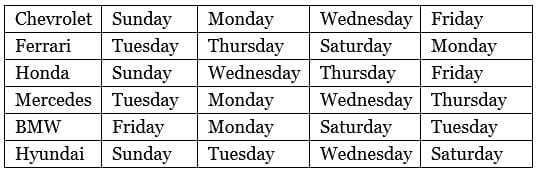The number of days in January and February 2008 on which he drove a car of brand Chevrolet, Ferrari, Honda, Mercedes, BMW and Hyundai is denoted by CH, FE, HO, ME, BM and HY respectively. It is also known that HO > ME > CH > BM > HY > FE. The following pie – chart gives details about the number of days in January and February 2008 on which he drove a car of brand Honda, BMW and Hyundai. (2014)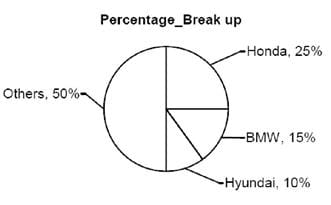Q. Given that the number of days in January 2008 on which Mr. Alfonso drove the car of brand Honda is the maximum. What is the number of days in February 2008 on which he drove the car of brand Honda?

Solution:

The total number of different days of the week in January and February 2008 are as follows:
Mondays: 8 (4 each in January and February)
Tuesdays: 9 (5 in January and 4 in February)
Wednesdays: 9 (5 in January and 4 in February)
Thursdays: 9 (5 in January and 4 in February)
Fridays: 9 (4 in January and 5 in February)
Saturdays: 8 (4 each in January and February)
Sundays: 8 (4 each in January and February)
Total number of days in January and February 2008 = 60 days
Total number of days on which he drove the car of brand
Honda = 25% of 60 = 60 x 25 / 100 = 15 days
Total number of days on which he drove the car of brand
BMW = 15% of 60 = 60 x 15 / 100 = 9 days
Total number of days on which he drove the car of brand
Hyundai = 10% of 60 = 60 x 10 / 100 = 6 days.
Here, HO > ME > CH > BM > HY > FE (given)
So,we get that 15 > ME > CH > 9 > 6 > FE.
Then, ME + CH + FE= 60 – (15 + 9 + 6) = 30 days
Here that the number of days on which Mr. Alfonso drove the car of brand in January 2008 is the maximum.
Now, the car of brand Honda is only driven on either of the three days of any week, i.e. Monday, Tuesdays and Saturdays.
Total number of Mondays, Tuesdays and Saturdays in January 2008
= 4 + 5 + 4 = 13 days
Total number of days in February 2008 on which he drove the car of brand Honda is = 15 – 13 = 2 days

QUESTION: 2

### Directions for Questions: Answer the questions on the basis of the information given below. Mr. Alfonso has six cars such that each car is of a different brand. The cars with Mr. Alfonso are of the six brands Chevrolet, Ferrari, Honda, Mercedes, BMW and Hyundai. In the months of January and February in the year 2008, Mr. Alfonso drove exactly one car each day. The following table gives details about the days in January and February 2008 on which he did not drive a car of each of the given six brands. Given that January 1, 2008 was a Tuesday.The number of days in January and February 2008 on which he drove a car of brand Chevrolet, Ferrari, Honda, Mercedes, BMW and Hyundai is denoted by CH, FE, HO, ME, BM and HY respectively. It is also known that HO > ME > CH > BM > HY > FE. The following pie – chart gives details about the number of days in January and February 2008 on which he drove a car of brand Honda, BMW and Hyundai. (2014)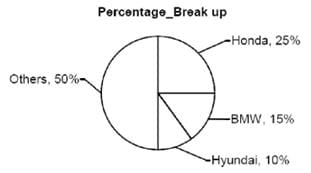Q. If Mr. Alfonso drove the car of brand Chevrolet on all possible Saturdays in January 2008 and all possible Thursdays in January 2008 and February 2008, then what is the number of days on which he drove Ferrari in the given two months?

Solution:

The total number of different days of the week in January and February 2008 are as follows:
Mondays: 8 (4 each in January and February)
Tuesdays: 9 (5 in January and 4 in February)
Wednesdays: 9 (5 in January and 4 in February)
Thursdays: 9 (5 in January and 4 in February)
Fridays: 9 (4 in January and 5 in February)
Saturdays: 8 (4 each in January and February)
Sundays: 8 (4 each in January and February)
Total number of days in January and February 2008 = 60 days
Total number of days on which he drove the car of brand Honda = 25% of 60 = 60 x 25 / 100 = 15 days
Total number of days on which he drove the car of brand BMW = 15% of 60 = 60 x 15 / 100 = 9 days
Total number of days on which he drove the car of brand
Hyundai = 10% of 60 = 60 x 10 / 100 = 6 days.
Here, HO > ME > CH > BM > HY > FE (given) So,we get that 15 > ME > CH > 9 > 6 > FE.
Then, ME + CH + FE= 60 – (15 + 9 + 6) = 30 days
Mr. Alfonso drove Chevrolet on all possible Saturdays in January 2008 i.e. 4 days and all possible Thursdays in January 2008 and February 2008 i.e. 9 days.
⇒ He drove Chevrolet on 13 days and Mercedes on 14 days as 15 > ME > CH
⇒ Number of days on which he drove Ferrari = 30 – (13 + 14) = 3 days.

QUESTION: 3

### Directions for Questions: Answer the questions on the basis of the information given below. Mr. Alfonso has six cars such that each car is of a different brand. The cars with Mr. Alfonso are of the six brands Chevrolet, Ferrari, Honda, Mercedes, BMW and Hyundai. In the months of January and February in the year 2008, Mr. Alfonso drove exactly one car each day. The following table gives details about the days in January and February 2008 on which he did not drive a car of each of the given six brands. Given that January 1, 2008 was a Tuesday.The number of days in January and February 2008 on which he drove a car of brand Chevrolet, Ferrari, Honda, Mercedes, BMW and Hyundai is denoted by CH, FE, HO, ME, BM and HY respectively. It is also known that HO > ME > CH > BM > HY > FE. The following pie – chart gives details about the number of days in January and February 2008 on which he drove a car of brand Honda, BMW and Hyundai. (2014)Q. What is the difference between the maximum and minimum possible number of days on which Mr. Alfonso drove the car of brand Mercedes in the given two months?

Solution:

The total number of different days of the week in January and February 2008 are as follows:
Mondays: 8 (4 each in January and February)
Tuesdays: 9 (5 in January and 4 in February)
Wednesdays: 9 (5 in January and 4 in February)
Thursdays: 9 (5 in January and 4 in February)
Fridays: 9 (4 in January and 5 in February)
Saturdays: 8 (4 each in January and February)
Sundays: 8 (4 each in January and February)
Total number of days in January and February 2008 = 60 days
Total number of days on which he drove the car of brand Honda = 25% of 60 = 60 x 25 / 100 = 15 days
Total number of days on which he drove the car of brand BMW = 15% of 60 = 60 x 15 / 100 = 9 days
Total number of days on which he drove the car of brand
Hyundai = 10% of 60 = 60 x 10 / 100 = 6 days.
Here, HO > ME > CH > BM > HY > FE (given) So,we get that 15 > ME > CH > 9 > 6 > FE.
Then, ME + CH + FE= 60 – (15 + 9 + 6) = 30 days
Maximum number of days on which Mr. Alfonso can drove the Mercedes in two months = 14 days
To minimize the number of number of days when he drove Mercedes in the given two months, we will maximize the number of days on which he drove Ferrari and Chevrolet.
He could drove Ferrari for a maximum of 5 days as FE < 6
⇒ ME + CH = 25 and ME > CH
⇒The minimum value of ME =13 days Difference between maximum and minimum value of ME = 14 – 13 = 1 day

QUESTION: 4

Directions for Questions: Answer the questions on the basis of the information given below.
The subject wise breakup of the marks obtained by 4 students in 5 subjects during their board examination is given below. Assume that all subjects carry equal maximum marks unless specified.

(2014)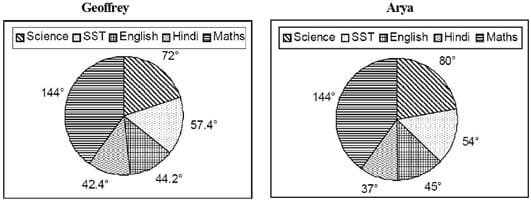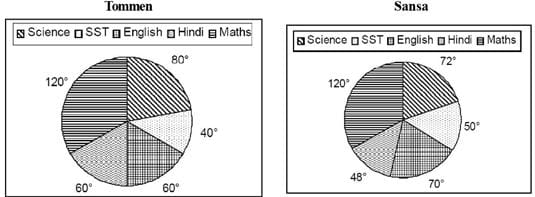Q. If the minimum percentage required to pass in any subject is 33.33 and it is also known that Arya passed in all the subjects, then what can be the minimum percentage that she can score in English?

Solution:

∵ Minimum percentage required to pass in a subject = 33.33%
Thus, 40° out of 360° represents 33.33%
⇒ 60° represents 50%.
∴ So, minimum percentage scored by Arya = 50%.

QUESTION: 5

Directions for Questions: Answer the questions on the basis of the information given below.
The subject wise breakup of the marks obtained by 4 students in 5 subjects during their board examination is given below. Assume that all subjects carry equal maximum marks unless specified.

(2014)Q. If the pattern of the examination is changed in such a way that the maximum marks for Maths is double of the maximum marks for any other subject (the breakup of marks remaining unchanged), then what is the ratio of maximum marks, in all the subjects put together, which Geoffrey can score to the maximum marks, in all the subjects put together, which Tommen can score?

Solution:

Let maximum marks for each subject other than maths. = 100
∴ Maximum marks in maths = 200
For Geoffrey, 144° = 200 marks
⇒ 360° = 500 marks.
For Tommen, if 120° = 200 marks
then 72° > 100 marks,
So, this is not possible, then
∴ For Tommen, 72° = 100 marks
⇒ 360° = 500 marks
∴ Ratio of maximum marks, in all the subjects put together, by Geoffrey and Tommen = 1 : 1.

QUESTION: 6

Directions for Questions: Answer the questions on the basis of the information given below.
The subject wise breakup of the marks obtained by 4 students in 5 subjects during their board examination is given below. Assume that all subjects carry equal maximum marks unless specified.

(2014)Q. If the pattern of the examination is changed in such a way that the maximum marks for Maths is double of the maximum marks for any other subject (the breakup of marks remaining unchanged), then what is the ratio of maximum marks, in all the subjects put together, which Sansa can score after the change in pattern and before the change in pattern?

Solution:

Before the change in pattern of examination
For maths:
∵ 144° = 100 marks
⇒ ∴ 360° = 250 marks.
After the change in pattern of examinaiton,
80° = 100 marks
⇒ 360° = 450 marks
∴ Required ratio = 450 : 250 = 9 : 5.

QUESTION: 7

Directions for Questions: Answer the questions on the basis of the information given below.
The subject wise breakup of the marks obtained by 4 students in 5 subjects during their board examination is given below. Assume that all subjects carry equal maximum marks unless specified.

(2014)Q. If the marks scored by Geoffrey in Maths is maximum possible then what is the average of percentage marks scored by him in all the subjects?

Solution:

According to question,
144° = 100 marks
⇒ 360° = 250 marks
∴ Average percentage = 250 / 500 x 100
Marks scored by Geoffrey = 50%.

QUESTION: 8

Directions for Questions: Answer the questions on the basis of the information given below.
The pie charts given below show the distribution of the number of athletes sent by three countries to take part in eight different games in the recently held Olympics. The numbers of athletes sent by USA, China and Russia were in the ratio 8 : 11 : 5. It is also known that the total number of athletes sent by the three countries put together was 2400.

(2013)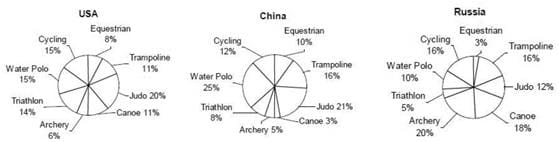Q. The female to male ratio for Cycling athletes from Russia and USA was 1 : 19 and 4 : 1 respectively. If the number of female Cycling athletes was equal to the number of male Cycling athletes for the three countries put together, then what was the number of female Cycling athletes from China?

Solution:

The total number of athelets sent by:
USA = 2400 × 8 / 24 = 800 athletes
China = 2400 × 11 / 24 = 1100 athletes
Russia = 2400 × 5 /24 = 500 athletes
Total number of Cycling athelets sent by the three countries together = 0.15 × 800 + 0.12 × 1100 + 0.16 × 500 = 332
∴ Total number of female cycling athletes sent by the three countries togeter = 166
Number of female cycling athletes sent by Russia
= 1 / 20 × (0.16 × 500) = 4
Number of female cycling atheletes sent by USA
= 4 / 5 × (0.15 × 800) = 96
Hence, the number of female cycling athletes sent by China =166 – (4 + 96) = 66.

QUESTION: 9

Directions for Questions: Answer the questions on the basis of the information given below.
The pie charts given below show the distribution of the number of athletes sent by three countries to take part in eight different games in the recently held Olympics. The numbers of athletes sent by USA, China and Russia were in the ratio 8 : 11 : 5. It is also known that the total number of athletes sent by the three countries put together was 2400.

(2013)Q. What was the absolute difference between the maximum number of athletes sent by China for a game and the minimum number of athletes sent by Russia for a game?

Solution:

The total number of athelets sent by:
USA = 2400 × 8 / 24 = 800 athletes
China = 2400 × 11 / 24 = 1100 athletes
Russia = 2400 × 5 /24 = 500 athletes
Maximum number of athletes sent by China for a game = 0.25 × 1100 = 275
Minimum number of athletes sent by Russia for a game = 0.03 × 500 = 15
∴ The required difference = 275 – 15 = 260

QUESTION: 10

Directions for Questions: Answer the questions on the basis of the information given below.
The pie charts given below show the distribution of the number of athletes sent by three countries to take part in eight different games in the recently held Olympics. The numbers of athletes sent by USA, China and Russia were in the ratio 8 : 11 : 5. It is also known that the total number of athletes sent by the three countries put together was 2400.

(2013)Q. The number of Water Polo athletes constituted what percent of the total number of athletes sent by the three countries put together?

Solution:

The total number of athelets sent by:
USA = 2400 × 8 / 24 = 800 athletes
China = 2400 × 11 / 24 = 1100 athletes
Russia = 2400 × 5 /24 = 500 athletes
The total number of Water Polo athletes sent by the three countries put together = 0.15 × 800 + 0.25 × 1100 + 0.10 × 500
= 120 + 275 + 50 = 445
∴ Total number of athletes sent by three countries = 2400
So, the required percentage
= 445 / 2400 x 100 = 18.54

QUESTION: 11

Directions for Questions: Answer the following questions on the basis of the information given below.
The following table gives the sales turnover (in crore ₹) of the five major detergent brands A, B, C, D, E and others in the 4 regions East, West, North and South of India. The data is for the years 2008 and 2009. The pie chart gives the breakup of the sales of the same brands in terms of the number of units sold. The total number of units sold for 2008 and 2009 was the same.

(2012)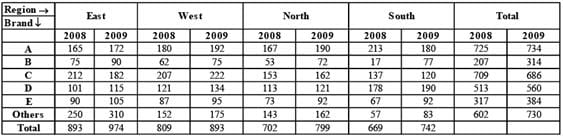Volume-wise Breakup of Brands All India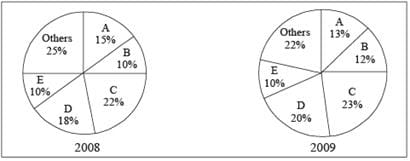Q. What is the total sales turnover (in crore ₹) of the detergent market in India in the year 2009?

Solution:

Total sales turnover of the detergent in East region of India in the year 2009 = 974 crores.
Total sales turnover of the detergent in West region of India in the year 2009 = 893 crores.
Total sales turnover of the detergent in North region of India in the year 2009 = 799 crores.
Total sales turnover of the detergent in South region of India in the year 2009 = 742 crores.
∴ Total sales turnover of all the detergent in 2009
⇒ 974 + 893 + 799 + 742 = 3408 crore.

QUESTION: 12

Directions for Questions: Answer the following questions on the basis of the information given below.
The following table gives the sales turnover (in crore ₹) of the five major detergent brands A, B, C, D, E and others in the 4 regions East, West, North and South of India. The data is for the years 2008 and 2009. The pie chart gives the breakup of the sales of the same brands in terms of the number of units sold. The total number of units sold for 2008 and 2009 was the same.

(2012)Volume-wise Breakup of Brands All IndiaQ. Which brand registered the maximum percentage increase in the price per unit in 2009?

Solution:

Percentage increase in price per unit in 2009.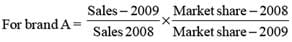⇒ 734 / 725 × 15 / 13 = 1.168%
For brand B ⇒ 314 / 207 x 10 / 12 = 1.264%
For brand C ⇒ 686 / 709 x 22 / 23 = 0.925%
For brand D ⇒ 560 / 513 x 18 / 20 = 0.982%
For brand E ⇒ 384 / 317 x 10 / 10 = 1.211%
So, brand B registered the maximum percentage increase in the price per unit in 2009.

QUESTION: 13

Directions for Questions: Answer the following questions on the basis of the information given below.
The following table gives the sales turnover (in crore ₹) of the five major detergent brands A, B, C, D, E and others in the 4 regions East, West, North and South of India. The data is for the years 2008 and 2009. The pie chart gives the breakup of the sales of the same brands in terms of the number of units sold. The total number of units sold for 2008 and 2009 was the same.

(2012)Volume-wise Breakup of Brands All IndiaQ. Which of the following statement(s) is/are true?
1. Exactly two brands could not register a growth in all the regions in 2009.
2. The number of units sold by B in 2009 has shown an increase of above 350% in South.
3. The number of units sold by C in 2009 was less than that sold by C in 2008.

Solution:

Let a total of 100N units be sold in both the years 2008 and 2009.
Statement 1:
A brand could not register growth in South and C brand could not register growth in both South and East in 2009.
So, Statement 1 is true
Statement 2:
Number of units sold by B in 2009 in South
= (12 / 100 x 100N) 77 / 314 = 2.94 N
Number of units sold by B in 2008 in South
= (10 / 100 x 100N) 17 / 207 = 0.82 N
Percentage increase
= (2.94 - 0.82 / 0.82) x 100 = 258%
So, Statement 2 is false.
Statement 3:
Number of units sold by C in 2009 (23N) is greater than the number of units sold by C in 2008 (22N).
So, Statement 3 is false.

QUESTION: 14

Directions for Questions: Answer the questions on the basis of the information given below.
The break-up of the financial budget of a country called Chaupatland for FY 2010-11 is represented by the pie charts given below. Pie chart A represents Budgeted Revenue and pie chart B represents Budgeted Expenditure.

(2010)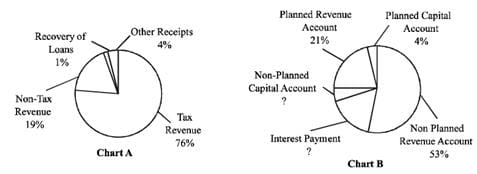Q. The difference between Budgeted Expenditure and Budgeted Revenue was what percentage of  Budgeted Revenue?

Solution:

The difference between Budgeted Expenditure and Budgeted Revenue when expressed as a percentage of Budgeted Revenue
= 625 / 500 x 100 = 125%

QUESTION: 15

Directions for Questions: Answer the questions on the basis of the information given below.
The break-up of the financial budget of a country called Chaupatland for FY 2010-11 is represented by the pie charts given below. Pie chart A represents Budgeted Revenue and pie chart B represents Budgeted Expenditure.

(2010)Q. By how much did the sum of Planned Revenue Account and Planned Capital Account exceed Non-Tax Revenue?

Solution:

The sum of Planned Revenue Account and Planned Capital Account (₹ 281.25 thousand crores) exceeded the Non-Tax Revenue (₹ 95 thousand crores) by ₹ 186.25 thousand crores.Use Code STAYHOME200 and get INR 200 additional OFF Use Coupon Code